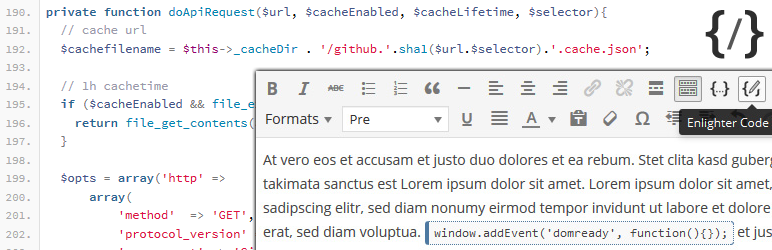This is a test page for code highlighting. I previously used the Crayon Syntax Highlighter for code formatting. Unfortunately this plugin hasn’t been updated in nearly four years and no longer appears to be maintained. I have chosen to replace it with the Code Syntax Block plugin.

I am also exploring the use of other plugins including the Enlighter – Customizable Syntax Highlighter plugin. Both support the new Gutenberg editor for WordPress. The Code Syntax Block plugin uses the Prism Syntax Highlighter at its core. Enlighter instead relies on the EnlighterJS javascript library to power it.Code Syntax Block

Code Syntax Block plugin extends the Gutenberg Block Editor by adding syntax highlighting to the core code block.

Code Syntax Block

### Code Syntax Block Examples:

#### Javascript:

``````class Timer extends React.Component {
constructor(props) {
super(props);
this.state = { seconds: 0 };
}

tick() {
this.setState(state => ({
seconds: state.seconds + 1
}));
}

componentDidMount() {
this.interval = setInterval(() => this.tick(), 1000);
}

componentWillUnmount() {
clearInterval(this.interval);
}

render() {
return (
<div>
Seconds: {this.state.seconds}
</div>
);
}
}

ReactDOM.render(
<Timer />,
document.getElementById('timer-example')
);``````

#### Ruby:

``````require("csim")
# The circuit here should simulate a level-triggered D flip-flop
D = SwitchBank.new
C = Pulser.new
N = NotGate.new
A1, A2 = AndGate.many(2)
NR1, NR2 = NorGate.many(2)
Q = LED.new(" Q")
Qnot = LED.new(" ~Q")

D.join(N)
N.join(A1)
D.join(A2)
C.join(A1)
C.join(A2)
A1.join(NR1)
A2.join(NR2)
NR1.join(NR2)
NR2.join(NR1)
LED.shush(true)
NR1.join(Q)
NR2.join(Qnot)
LED.shush(false)
vals = [ true, false, false, true, false ]
10.times do
3.times do
dval = vals.shift
vals.push(dval)
print "Input: ", dval, "\n"
D.value = dval
end
print "=== TIC ===\n"
C.pulse
end``````

#### Python:

``````from time import localtime

activities = {
8: 'Sleeping',
9: 'Commuting',
17: 'Working',
18: 'Commuting',
20: 'Eating',
22: 'Resting' }
time_now = localtime()
hour = time_now.tm_hour
for activity_time in sorted(activities.keys()):
if hour < activity_time:
print activities[activity_time]
break
else:
print 'Unknown, AFK or sleeping!'``````

#### PowerShell:

``````function Show-Calendar {
param(
[DateTime] \$start = [DateTime]::Today,
[DateTime] \$end = \$start,
\$firstDayOfWeek,
[int[]] \$highlightDay,
[string[]] \$highlightDate = [DateTime]::Today.ToString()
)

#actual code for the function goes here see the end of the topic for the complete code sample
}``````Enlighter – Customizable Syntax Highlighter

All-in-one Syntax Highlighting solution. Full Gutenberg and Classic Editor integration. Graphical theme customizer. Based on EnlighterJS.

Enlighter – Customizable Syntax Highlighter

### Enlighter Examples:

#### Javascript:

```class Timer extends React.Component {
constructor(props) {
super(props);
this.state = { seconds: 0 };
}

tick() {
this.setState(state => ({
seconds: state.seconds + 1
}));
}

componentDidMount() {
this.interval = setInterval(() => this.tick(), 1000);
}

componentWillUnmount() {
clearInterval(this.interval);
}

render() {
return (
<div>
Seconds: {this.state.seconds}
</div>
);
}
}

ReactDOM.render(
<Timer />,
document.getElementById('timer-example')
);```

#### Ruby:

```require("csim")
# The circuit here should simulate a level-triggered D flip-flop
D = SwitchBank.new
C = Pulser.new
N = NotGate.new
A1, A2 = AndGate.many(2)
NR1, NR2 = NorGate.many(2)
Q = LED.new(" Q")
Qnot = LED.new(" ~Q")

D.join(N)
N.join(A1)
D.join(A2)
C.join(A1)
C.join(A2)
A1.join(NR1)
A2.join(NR2)
NR1.join(NR2)
NR2.join(NR1)
LED.shush(true)
NR1.join(Q)
NR2.join(Qnot)
LED.shush(false)
vals = [ true, false, false, true, false ]
10.times do
3.times do
dval = vals.shift
vals.push(dval)
print "Input: ", dval, "\n"
D.value = dval
end
print "=== TIC ===\n"
C.pulse
end```

#### Python:

```from time import localtime

activities = {
8: 'Sleeping',
9: 'Commuting',
17: 'Working',
18: 'Commuting',
20: 'Eating',
22: 'Resting' }
time_now = localtime()
hour = time_now.tm_hour
for activity_time in sorted(activities.keys()):
if hour < activity_time:
print activities[activity_time]
break
else:
print 'Unknown, AFK or sleeping!'```

#### PowerShell:

Beta Version of Enlighter doesn’t appear to support the PowerShell language at this time.

```function Show-Calendar {
param(
[DateTime] \$start = [DateTime]::Today,
[DateTime] \$end = \$start,
\$firstDayOfWeek,
[int[]] \$highlightDay,
[string[]] \$highlightDate = [DateTime]::Today.ToString()
)

#actual code for the function goes here see the end of the topic for the complete code sample
}```

## Justin C.

Technology Enthusiast

I’m a Soldier by day and coder by night. A life long learner of cyber security, penetration testing, machine learning, circuit design, art, and photography.